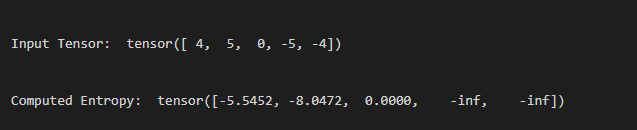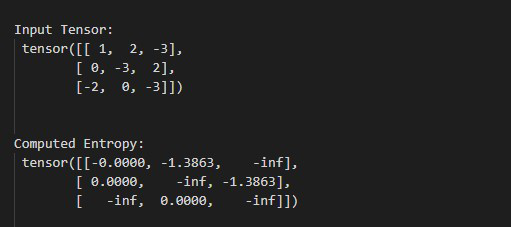# How to compute element-wise entropy of an input tensor in PyTorch

• Last Updated : 21 Apr, 2022

In this article, we are going to discuss how to compute the element-wise entropy of an input tensor in PyTorch, we can compute this by using torch.special.entr() method.

## torch.special.entr() method

torch.special.entr() method computes the element-wise entropy, This method accepts a tensor as input and returns a tensor with the element-wise entropy of the input tensor. if the element is zero, or negative then entropy is also zero, or negative infinity respectively. before moving further let’s see the syntax of the given method.

Syntax: torch.special.entr(tens)

Parameters:

• tens: This is our input tensor.

Returns: Returns the elements-wise entropy of an input tensor.

Example 1:

The following program is to understand how to compute the element-wise entropy of a 1D tensor.

## Python

 `# import torch libraries ` `import` `torch ` ` `  `# creating a 1D tensor ` `tens ``=` `torch.tensor([``4``, ``5``, ``0``, ``-``5``, ``-``4``]) ` ` `  `# Display tensor ` `print``(``"\n\nInput Tensor: "``, tens) ` ` `  `# compute the element-wise entropy of  ` `# input tensor ` `entr ``=` `torch.special.entr(tens) ` ` `  `# Display result ` `print``(``"\n\nComputed Entropy: "``, entr) `

Output:Example 2:

The following program is to know how to compute the element-wise entropy of a 2D tensor.

## Python

 `# import torch libraries ` `import` `torch ` ` `  `# creating a 2D tensor ` `tens ``=` `torch.tensor([[``1``, ``2``, ``-``3``], ` `                     ``[``0``, ``-``3``, ``2``], ` `                     ``[``-``2``, ``0``, ``-``3``]]) ` ` `  `# Display tensor ` `print``(``"\n Input Tensor: \n"``, tens) ` ` `  `# compute the element-wise entropy of ` `# input tensor ` `entr ``=` `torch.special.entr(tens) ` ` `  `# Display result ` `print``(``"\n Computed Entropy: \n"``, entr) `

Output:My Personal Notes arrow_drop_up
Recommended Articles
Page :